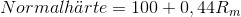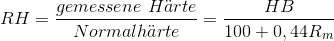## Relative hardness

Quality characteristics for flake graphite cast iron.

Relative hardness is the quotient of actually achieved hardness HB (measured with a 30 mm standard test bar, see Brinell hardness test) and the standard hardness calculated from the tensile strength which is calculated from Eq. 1 as follows:

Eq. 1:According to Eq. 2, the relative hardness is directly calculated from the measured Brinell hardness HB and the tensile strength Rm as follows:

Eq. 2:In general, cast iron with low relative hardness and a high degree of normality (q. v.) should be pursued.

Additional references:

Hardness test
Tensile strength/Hardness quotient